Questions from the forum
 Q: How can I display strings and numbers on a panel? A: Here's an example that uses a "digits" instruction to do that.   FONT* arial_font = "Arial#20";   PANEL* display_pan = {        pos_x = 100;        pos_y = 200;        layer = 15;        digits(10, 10, "Number of seconds: %.f", arial_font, 1, sys_seconds);        flags = visible; }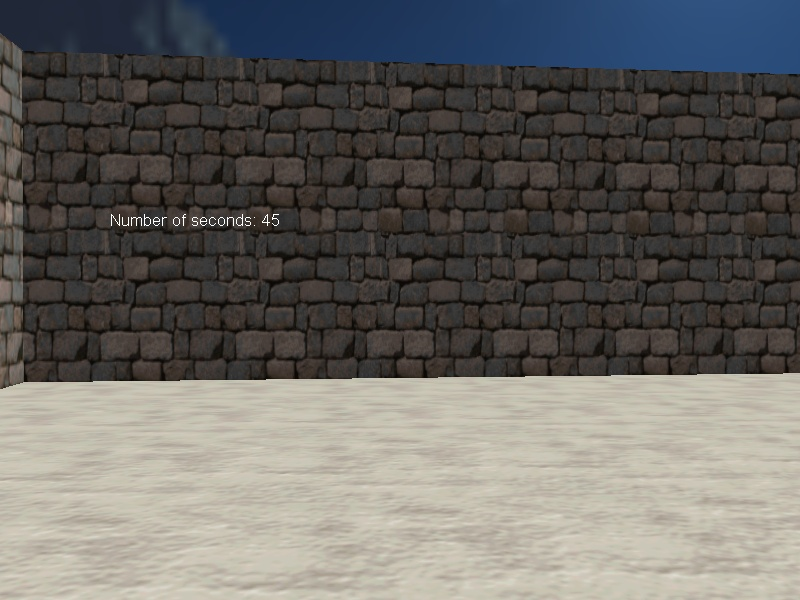Q: I'm making a 2.5D sidescroller game and I'd like to have an enemy that shoots towards the player if it comes close enough to it. How would I do this? A: There you go.   function move_bullet(); function remove_bullet();   function initialize_startup() {        fps_max = 75; // limit the frame rate to 75 fps         }   action players_code() // simple player action {                var movement_speed = 20;        VECTOR temp;        set (my, INVISIBLE);        player = my;        my.skill99 = 100; // the player starts with 100 health points        while (my.skill99 > 0) // this loop will run for as long as the player is alive        {                my.pan -= 7 * mouse_force.x * time_step;                vec_set (temp.x, my.x);                temp.z -= 10000;                temp.z = -c_trace (my.x, temp.x, IGNORE_ME | IGNORE_PASSABLE | USE_BOX) - 2;                temp.x = movement_speed * (key_w - key_s) * time_step;                temp.y = movement_speed * (key_a - key_d) * 0.6 * time_step;                c_move (my, temp.x, nullvector, IGNORE_PASSABLE | GLIDE);                camera.x = my.x;                camera.y = my.y;                camera.z = my.z + 50;                camera.pan = my.pan;                camera.tilt += 5 * mouse_force.y * time_step;                wait (1);        }        camera.roll = 20; // the player is dead here }   action simple_enemy() {        VECTOR temp;        while (!player) {wait (1);}        while (1)        {                // the player has come too close to the enemy                if (vec_dist (player.x, my.x) < 1000) // 1000 gives the enemy's range                {                        vec_set(temp, player.x);                        vec_sub(temp, my.x);                        vec_to_angle(my.pan, temp); // rotate the enemy towards the player                        // the enemy fires a bullet every 2 seconds (150 = 2 x 75 frames, the frame rate was limited to 75 fps)                        if ((total_frames % 150) == 1)                        {                                ent_create("bullet.mdl", my.x, move_bullet);                        }                }                        wait (1);        } }   function move_bullet() {        VECTOR bullet_speed; // this var will store the speed of the bullet        my.skill30 = 1; // I'm a bullet        // the bullet is sensitive to impact with other entities and to impact with level blocks        my.emask |= (ENABLE_IMPACT | ENABLE_ENTITY | ENABLE_BLOCK);        my.event = remove_bullet; // when it collides with something, its event function (remove_bullet) will run        my.pan = you.pan; // the bullet has the same pan        my.tilt = you.tilt; // and tilt with the enemy        bullet_speed.x = 50 * time_step; // adjust the speed of the bullet here        bullet_speed.y = 0; // the bullet doesn't move sideways        bullet_speed.z = 0; // or up / down on the z axis        // the loop will run for as long as the bullet exists (it isn't "null")        while (my)        {                // move the bullet ignoring its creator (the enemy)                c_move (my, bullet_speed, nullvector, IGNORE_YOU);                wait (1);        } }   function remove_bullet() // this function runs when the bullet collides with something {        wait (1); // wait a frame to be sure (don't trigger engine warnings)        if (you) // collided with an entity?        {                if (you == player) // collided with the player? Then let's do some damage!                        player.skill99 -= 10; // each shot takes 10 health points from the player        }        ent_remove (my); // and then remove the bullet }     Q: I am making a small simulator with a 2d top down view (country map and sea). What is the best way to implement zoom in / out using the mouse scrolling wheel? I'd like to have 5 levels of zoom: max, 3 intermediary stages, min. A: Here's a simple example.   // play with the values below - they depend on the actual size of your level var min_offset_x = -1000; // the level starts at about -1000 quants var max_offset_x = 1500; // and goes up to 1500 quants on the x axis in this example   var min_offset_y = -1200; // the level starts at about -1200 quants var max_offset_y = 1300; // and goes up to 1300 quants on the y axis in this example   var horizontal_speed = 10; // horizontal scrolling speed var vertical_speed = 8; // vertical scrolling speed   BMAP* pointer_tga = "pointer.tga";   function camera_startup() {        wait (-1);// wait until the level is loaded        camera.tilt = -60; // make the camera look downwards        camera.pan = 90; // set the proper pan angle        camera.x = (min_offset_x + max_offset_x) / 2; // set the initial camera position close to the center of the level        camera.y = (min_offset_y + max_offset_y) / 2; // on both axis        mouse_mode = 2;        mouse_map = pointer_tga;        camera.arc = 90; // set the default zoom factor        while (1)        {                vec_set(mouse_pos, mouse_cursor);                if ((mouse_pos.x < 1) && (camera.x > min_offset_x))                        camera.x -= horizontal_speed * time_step;                if ((mouse_pos.x > screen_size.x - 2) && (camera.x < max_offset_x))                        camera.x += horizontal_speed * time_step;                if ((mouse_pos.y > screen_size.y - 2) && (camera.y > min_offset_y))                        camera.y -= vertical_speed * time_step;                if ((mouse_pos.y < 1) && (camera.y < max_offset_y))                        camera.y += vertical_speed * time_step;                  if (mickey.z < -1) // the player has moved the mouse wheel because he / she wants to zoom in?                {                        camera.arc -= 30;                }                if (mickey.z > 1) // the player has moved the mouse wheel because he / she wants to zoom out?                        camera.arc += 30;                camera.arc = clamp(camera.arc, 30, 150); // each zoom step adds / subtracts 30 from camera.arc                wait (1);        } }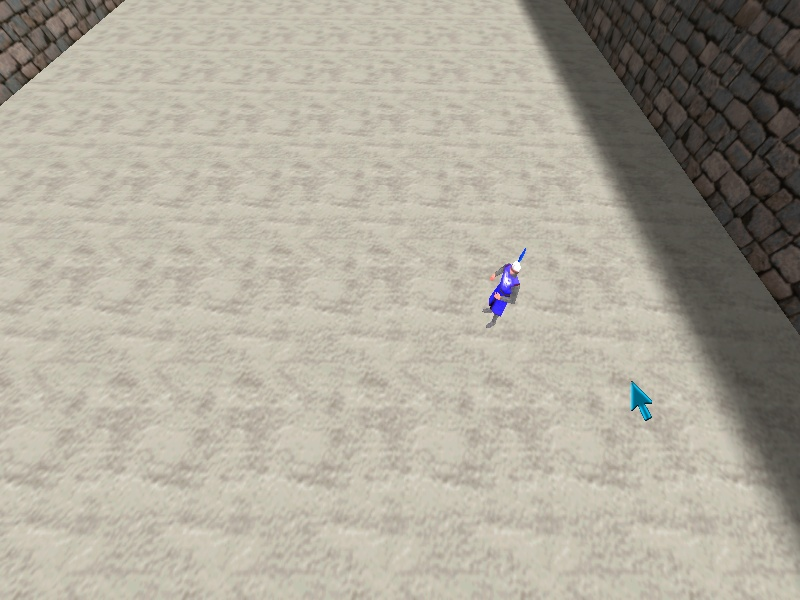Q: I want to create several models faster and faster, but I can't do it. Can anyone help? A: There you go:   var number_of_models = 100; // generate 100 models var pause_time = 200;   function move_ball() {        while (my.z < 10000)        {                my.z += 15 * time_step;                wait (1);        }        ent_remove(my); }   // attach this action to any model - it will become invisible action model_generator() {        set (my, PASSABLE | INVISIBLE);        wait (-5); // wait for 5 seconds        while (number_of_models > 0)        {                // use your own model file here                ent_create("ball.mdl", my.x, move_ball);                pause_time -= 15 * time_step;                pause_time = maxv(10, pause_time);                number_of_models -= 1;                wait (pause_time * time_step);        } }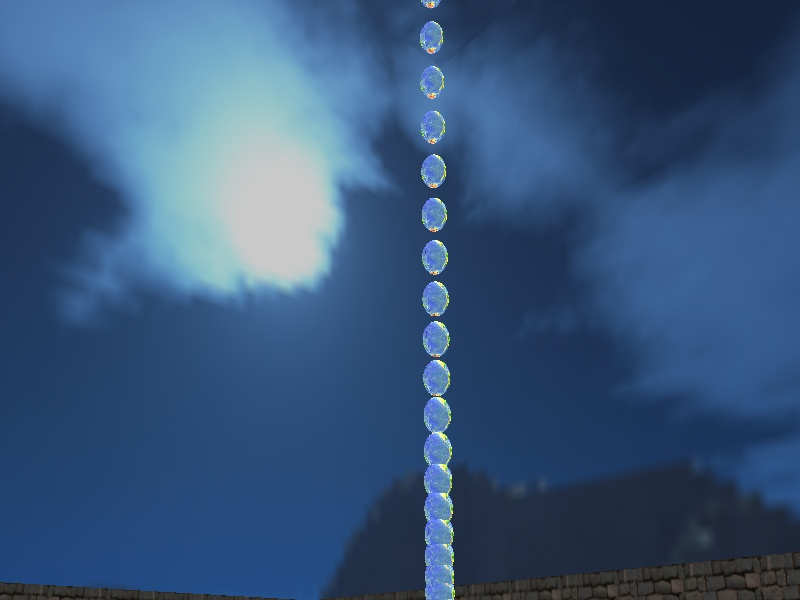Q: I need to create models in random positions, but I can't get this to work. A: Here's a simple, fully working example that generates 100 balls in random positions (x = -400... 400 quants, y = -500... 500 quants, z = 100) and moves each one of them up and down in a loop.   var number_of_balls = 100; // generate 100 balls   VECTOR ball_position;   function moving_ball() {        while (1)        {                my.z += 5 * sin(0.05 * total_ticks) * time_step;                wait (1);        } }   function balls_startup() {        wait (-3); // wait for 3 seconds        while (number_of_balls > 0)        {                ball_position.x = 400 - random(800);                ball_position.y = 500 - random(1000);                ball_position.z = 100;                // use your own model file here                ent_create("ball.mdl", ball_position.x, moving_ball);                number_of_balls -= 1;                wait (1);        } }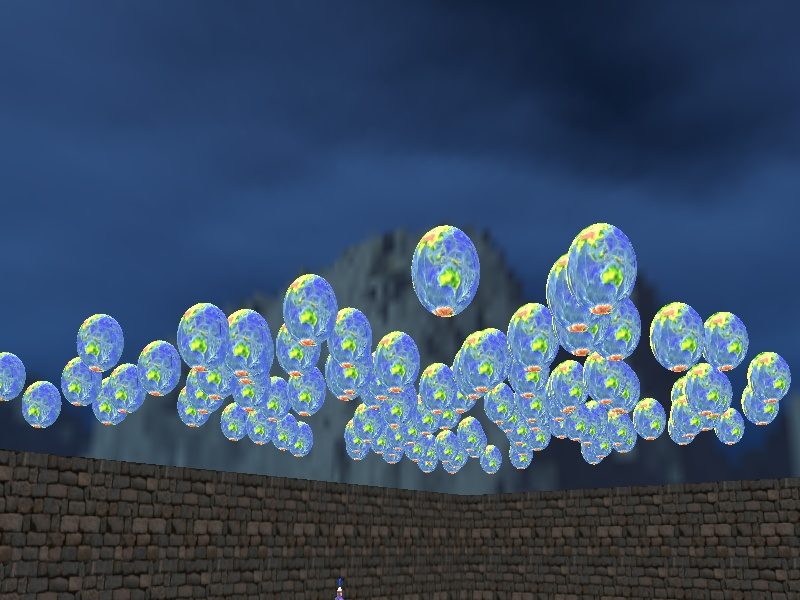Q: I'd like to have an UFO hover above player's head, dropping bombs when it manages to get right above it. Can you help? A: Here's an example.   function framerate_startup() {        fps_max = 75; // limit the frame rate to 75 fps }   function remove_bomb() {        wait (1); // wait a frame to be sure (don't trigger engine warnings)        ent_remove (my); // and then remove the bomb }   function move_bomb() {        my.emask |= (ENABLE_IMPACT | ENABLE_ENTITY | ENABLE_BLOCK);        my.event = remove_bomb;        while (my)        {                // the bombs move downwards with the speed given by 10                c_move(my, vector(0, 0, -10 * time_step), nullvector, IGNORE_PASSABLE);                wait (1);        }                         }   action evil_ufo() {        while (!player) {wait (1);} // wait until the player model is loaded        while (1)        {                if (abs(player.x - my.x) > 1)                        my.x += (player.x - my.x) * 0.03 * time_step; // 0.03 = tracking speed along the x axis                if (abs(player.y - my.y) > 1)                        my.y += (player.y - my.y) * 0.03 * time_step; // 0.03 = tracking speed along the y axis                // the ufo is close enough to the player? Then let's drop some bombs!                if ((abs(player.x - my.x) < 50) && (abs(player.y - my.y) < 50))                {                        if ((total_frames % 150) == 1) // drop a bomb every 2 seconds (75 fps x 2)                        {                                ent_create ("bomb.mdl", vector(my.x, my.y, my.z - 40), move_bomb);                        }                }                wait(1);        } }     Q: I use a separate VIEW* for my cut scenes. I'd like this view to activate and point towards an entity (a spaceship) 3 seconds after the player has come close enough to it. How can I do that? A: Use this snippet.   VIEW* cutscene_camera = {        pos_x = 0;        pos_y = 0;        layer = 10; // appears on top of the default camera view }   function init_startup() {        cutscene_camera.size_x = screen_size.x;        cutscene_camera.size_y = screen_size.y;        while (1)        {                                wait (1);        } }   action my_spaceship() {        VECTOR temp;        while (!player) {wait (1);}        vec_set(temp, my.x);        vec_sub(temp, cutscene_camera.x);        vec_to_angle(cutscene_camera.pan, temp); // the cut scene camera was rotated towards the spaceship already, but it isn't visible yet        while (vec_dist (player.x, my.x) > 300) {wait (1);} // wait until the player comes close enough to the player        wait (-3); // wait for 3 more seconds        set(cutscene_camera, SHOW); // now display the cut scene camera         }     Q: I want to create a car that has its wheels attached at runtime. How can I do that? A: Here's a fully functional example for a car that moves in a circle.   STRING* wheel_mdl = "wheel.mdl";   function front_left_wheel() {        VECTOR wheel_offset;        set (my, PASSABLE);        while (1)        {                vec_set(wheel_offset, vector(43, -20, -15));                vec_rotate(wheel_offset, you.pan);                vec_add(wheel_offset.x, you.x);                vec_set(my.x, wheel_offset.x);                my.pan = you.pan;                my.roll = you.roll;                wait (1);        } }   function front_right_wheel() {        VECTOR wheel_offset;        set (my, PASSABLE);        while (1)        {                vec_set(wheel_offset, vector(43, 20, -15));                vec_rotate(wheel_offset, you.pan);                vec_add(wheel_offset.x, you.x);                vec_set(my.x, wheel_offset.x);                my.pan = you.pan;                my.roll = you.roll;                wait (1);        } }   function back_left_wheel() {        VECTOR wheel_offset;        set (my, PASSABLE);        while (1)        {                vec_set(wheel_offset, vector(-32, -20, -15));                vec_rotate(wheel_offset, you.pan);                vec_add(wheel_offset.x, you.x);                vec_set(my.x, wheel_offset.x);                my.pan = you.pan;                my.roll = you.roll;                wait (1);        } }   function back_right_wheel() {        VECTOR wheel_offset;        set (my, PASSABLE);        while (1)        {                vec_set(wheel_offset, vector(-32, 20, -15));                vec_rotate(wheel_offset, you.pan);                vec_add(wheel_offset.x, you.x);                vec_set(my.x, wheel_offset.x);                my.pan = you.pan;                my.roll = you.roll;                wait (1);        } }   action car_body() // attach this action to your car body model {        my.ambient = -40;        ent_create(wheel_mdl, my.x, front_left_wheel); // create the front left wheel model        ent_create(wheel_mdl, my.x, front_right_wheel); // create the front right wheel model        ent_create(wheel_mdl, my.x, back_left_wheel); // create the back left wheel model        ent_create(wheel_mdl, my.x, back_right_wheel); // create the back right wheel model        while (1)        {                // simple snippet, moves the car in a circle                c_move (my, vector(5 * time_step, 0, 0), nullvector, IGNORE_PASSABLE | GLIDE);                my.pan += 2 * time_step; // 2 sets the radius of the rotation circle                             wait (1);        } }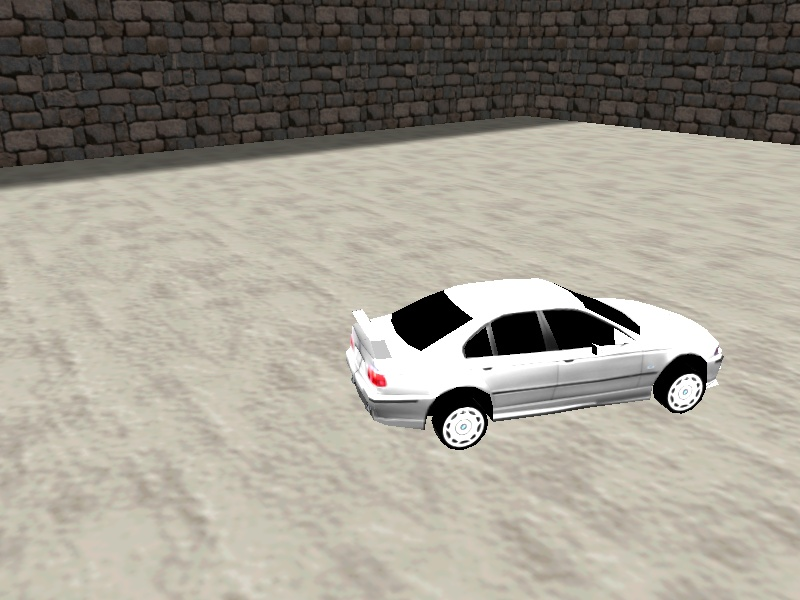Q: How can I remove a button from a panel after I've clicked it? I only need to press it once. A: Create the "button" as a separate panel; use the code below as a base for your code.   BMAP* main_pcx = "main.pcx"; BMAP* button_pcx = "button.pcx"; BMAP* pointer_tga = "pointer.tga";   function remove_button();   PANEL* main_pan = {        layer = 15;        pos_x = 300;        pos_y = 200;        bmap = main_pcx;        flags = SHOW; }   PANEL* button_pan = {        layer = 20;        pos_x = 320;        pos_y = 210;        bmap = button_pcx;        flags = SHOW;        on_click = remove_button; }   function mouse_startup() {         mouse_mode = 2;        mouse_map = pointer_tga;        while (1)        {                 vec_set(mouse_pos, mouse_cursor);                wait(1);        } }   function remove_button() {        while(mouse_left) {wait (1);}        reset(button_pan, SHOW);                beep(); // do your one-time operation here }     Q: I'd like to have a looping soundtrack in my main menu. When the player presses a button, the loop should fade out and the game should start. A: Here's a snippet that does what you want.   var loop_volume = 100; var soundtrack_handle;   BMAP* main_pcx = "main.pcx"; BMAP* pointer_tga = "pointer.tga"; BMAP* button1_pcx = "button1.pcx"; BMAP* button2_pcx = "button2.pcx";   function fade_music();   PANEL* main_pan = {        layer = 15;        pos_x = 300;        pos_y = 200;        bmap = main_pcx;        button(40, 10, button2_pcx, button2_pcx, button1_pcx, fade_music, NULL, NULL);        flags = SHOW; }   function mouse_startup() {         soundtrack_handle = media_loop("soundtrack.wav", NULL, loop_volume);        mouse_mode = 2;        mouse_map = pointer_tga;        while (1)        {                 vec_set(mouse_pos, mouse_cursor);                wait(1);        } }   function fade_music() {        while (mouse_left) {wait (1);} // wait until the mouse button is released        while (loop_volume > 1)        {                media_tune(soundtrack_handle, loop_volume, 0, 0);                loop_volume -= 1.5 * time_step; // 1.5 = fading speed                wait (1);        }        snd_stop (soundtrack_handle);        mouse_mode = 0; // hide the mouse pointer        reset(main_pan, VISIBLE); // hide the main panel        // start your game here: load a level, etc }